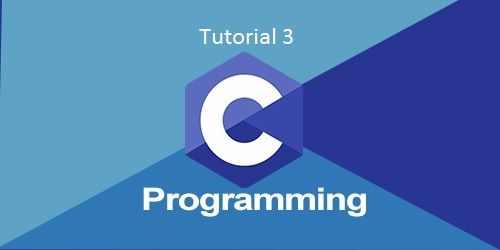# C programming language tutorial #3Image source – google |image by- nareshit.com

## Introduction:

In this third tutorial we learn some main programs which is very important for c programming language.

And this is last tutorial of the c  programming language tutorial series this series is basic tutorial of c programming language.

And after this last tutorial, you are able to do very complex program of c programming due to these basic tutorials you are able to start learning advance level c programming it will help you very much in future learning of c programming.

### (1)C program to swap two numbers:

#include<stdio.h>

#include<conio.h>

Void main()

{

Int a,b,c;

Printf(“enter the value of a:”);

Scanf(“%d”,&a);

Printf(“enter the value of b:”);

Scanf(“%d",&b);

Printf(“before swap\n”);

Printf(“enter int 1=%d\n”,a);

Printf(“enter int 2=%d\n”,b);

c=a;

b=c;

a=b;

printf(“after swap\n”);

printf(“int 1=%d\n”,a);

printf(“int2=%d\n”,b);

getch();

}

Output:

Enter the value of a=10

Enter the value of b=20

Before swap

Int a=10

Int b=20

After swap

Int a=20

Int b=10

Description:

In this program, all other things is same as first two tutorials but the additional logic are as below:

c=a;

a=b;

b=c;

in this consider that the a=10 and b=20 than c=0 and as logic c=a so now the c=10 and a=0.

Then a=b so now the a=20 and b=0.

Then b=c so now the b=10 and c=0.

So after this logic at the end, you get value of a=20 and b=10.

### (2)C program to convert distance in centimeter to feet and inches.

#include<stdio.h>

#include<conio.h>

Void main()

{

Int e,f,Inc,cen,feet;

Printf(“enter length in centimeter:”);

Scanf(“%d”,c);

Inc=C/2.54;

Feet=C/30.48;

Printf(“length in inch is: %d\n”,=inc);

Printf(“length in feet is: %d\n”,feet);

getch();

}

Output:

Enter a length in centimeter:100

Length in inch is: 39

Length in feet is: 3

Description:

In this program we say user to enter the length in centimeter and then we use a formula to convert the length from centimeter to inch and feet.

Inc=C/2.54;

Feet=C/30.48;

So this is a formula to convert the length from centimeter to inch and feet.

(3)c program to convert upper case letter to lower case latter

#include<stdio.h>

#include<conio.h>

int main()

{

Char s;

Printf(“enter word”);

Gets(s);

For(i=0;s[i]!=’\0’;i++)

{

If(s>=’a’&&s[i]<=’z’)

{

s[i]=s[i]-32;

}

}

Printf(“word in upper case =%s”,s);

Return 0;

}

Output:

Enter word big

Word in upper case=BIG

Description:

In this program

For(i=0;s[i]!=’\0’;i++)

{

If(s>=’a’&&s[i]<=’z’)

{

s[i]=s[i]-32;

this is a logic in this we used for loop and we put the condition and final logic to understand this we need to learn loops in c programing.

### What is loop in c program:

There are 3 loops in c programming while loop,do-while loop, for loop In this loop we put some conditions so the program which is an inside loop will repeat till the condition is satisfied.

When condition is satisfied then the compiler exit the loop and stop repeating the program or logic inside the loop.

Type of Loops in c programming :

There is three types of loop in c programing

(1)for loop

(2)while loop

(3)do-while loop

#### (1)for loop:

the for loop is a easiest loop in c programming by using this loop we can do any program which is done by using other two loops.

Syntax:

for(value of int;condition;increment or decrement)

{

Body of loop/program which we want to repeat ;

}

Ex. program of for loop

Print 1 to 10 numbers:

#include<stdio.h>

#include<conio.h>

Void main()

{

Int I;

for(I = 1; I <= 10; i++)

{

Printf(“%d\t”,i);

}

}

Output:

1 2 3 4 5 6 7 8 9 10

#### While loop:

In while loop  we put condition before program and then the assigning of value and increment and decrement is decide inside the program.

Syntax:

While(condition)

{

A program which we want to repeat

Variable increment or decrement ;

}

Ex. program

We take same program which we will done in for loop

Program to print 1 to 10 number:

#include<stdio.h>

#include<conio.h>

Void main()

{

Int I;

While(x <= 10)

{

Printf(“%d\t”,x);

X++;

}

}

Output:

1 2 3 4 5 6 7 8 9 10

#### do-while loop:

in this loop the condition will come after the program so first, the program will be executed and then condition will check.

Syntax:

do

{

Program which we want to repeat

}

While(condition)

Ex. program:

Program to print 1 to 10 numbers using do while loop

#include<stdio.h>

#include<conio.h>

Void main()

{

Int i;

i=1;

do

{

Printf(“%d\t”,i);

i++;

}

While(i<=10);

}

Output:

1 2 3 4 5 6 7 8 9 10

Example program of while loop

C program to find the numbers which are divisible by 3 in between the numbers 10 and 20:

#include<stdio.h>

#include<conio.h>

Void main()
{

Int I = 10;

While (I <= 20)

{

If(i%3==0)

{

Printf(“%d\n”,I);

}

I++;

}

}

getch()

}

Out put:

3,6,9,12,15,18

Explanation:

So in this program, we use  while loop and the logic is first we assign value to “I” integer the value is 10 so the I = 10 from start of program.

Then we use while loop in this we put a condition I <=20 so now if value of “I” will increase from 20 then loop is over and then loop will not execute.

And then we use one other condition which is “if” condition so when numbers are divisible by 3 then it will be printed on output screen and if number is not divisible by 3 then it will not be printed on output screen.

### Conclusion:

In this tutorial, we learn that what is a loop in c programming, how to use a loop in various programs, and how to implement many complex c programs   using looping.